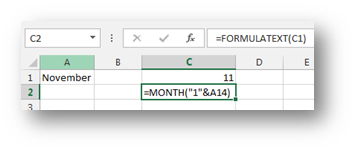# Ms Excel MONTH function madness

Published on
8,360 Points
2,060 Views
3 Endorsements
Last Modified:
Approved
Here's a very little known use of the commonly used Excel function MONTH which in it's essence returns the month number of the date or serial number it is given.

I guess we are all familiar with the MONTH function in Excel but let us take some time to go through the process. If you give it a date, well not the date exactlly but a serial number that belongs to a certain date, it will return the whole number value corresponding to that month. So very important, it takes a number (serial number belonging to the date) and it returns a number. So far so good. Now here's the strange thing…

I will use an example to describe this. If for example cell A1 contains a name of a month (let's say November) and you want to get the month number out of it. Now some might say VLOOKUP, add a second table of a number series from 1 to 12 and the corresponding month names in the second column. Now they would be spot on but here what you can also get the same result. You can write the following function =MONTH("1"&A1) and as you press enter, and just like that, you get 11. Wow!The reason why this works is the same reason for which the Excel will return a date if you write 1November in a Cell. It will understand that as the 1st of November in the current year, or a numeric value corespondidng to that date so the MONTH function has no problem returning the month number.

But since the 19March (for Example)  is not a very commonly used  way for a date input in Excel, it is not considered (or simply nobody knows) that you can actually use various date functions with this kind of date input.

This is just an example of the MONTH function but all other date function would work just as well.
3
Ask questions about what you read
If you have a question about something within an article, you can receive help directly from the article author. Experts Exchange article authors are available to answer questions and further the discussion.
Get 7 days free